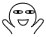GTNH由于添加了一些和工业2作物联动的mod，加入了许多新的作物，通过这些作物，甚至可以实现的矿物自动生产和UU物质的廉价生产。可以说是非常OP了。

```robot.forward()
robot.back()
robot.turnLeft()
robot.turnRight()
```

```local robot = require("robot")

local nowFacing = 1
local nowPos = {0, 0}
local savedFacing = 0
local savedPos = {0, 0}

local function getFacing()
return nowFacing
end

local function getPos()
return nowPos
end

local function turnTo(facing)
local delta = (facing - nowFacing) % 4
nowFacing = facing
if delta <= 2 then
for i = 1, delta do
robot.turnRight()
end
else
for i = 1, 4 - delta do
robot.turnLeft()
end
end
end

local function turningDelta(facing)
local delta = (facing - nowFacing) % 4
if delta <= 2 then
return delta
else
return 4-delta
end
end

local function go(pos)
if nowPos == pos and nowPos == pos then
return
end

-- find path
local posDelta = {pos-nowPos, pos-nowPos}
local path = {}

if posDelta > 0 then
path[#path+1] = {2, posDelta}
elseif posDelta < 0 then
path[#path+1] = {4, -posDelta}
end

if posDelta > 0 then
path[#path+1] = {1, posDelta}
elseif posDelta < 0 then
path[#path+1] = {3, -posDelta}
end

-- optimal first turn
if #path == 2 and turningDelta(path) < turningDelta(path) then
path, path = path, path
end

for i=1, #path do
turnTo(path[i])
for _=1, path[i] do
robot.forward()
end
end

nowPos = pos
end

local function save()
savedFacing = nowFacing
savedPos = nowPos
end

local function resume()
if savedFacing == 0 then
return
end
go(savedPos)
turnTo(savedFacing)
end

return {
getFacing = getFacing,
getPos = getPos,
turnTo = turnTo,
go = go,
save = save,
resume = resume
}
```

```-- 加载库 (请确保如上代码已保存为gps.lua)
-- 加载库的位置为初始位置，面向的方向为y轴正方向。
gps = require("gps.lua")
-- 前往坐标{2, 3}
gps.go({2, 3})
-- 前往坐标{4, 5}
gps.go({4, 5})
-- 保存当前位置和朝向
gps.save()
-- 前往坐标{0, 0}
gps.go({0, 0})
-- 前往保存的坐标和朝向
gps.resume()
```

## 评论

1.LiChen
2年前
2021-8-21 9:29:16

还有吗 还有吗•博主
LiChen
2年前
2021-8-28 15:57:46

半年前就做完了整个项目了，但是懒得写博文https://github.com/xyqyear/auto-crossbreeding

## 发送评论编辑评论

|´・ω・)ノ
ヾ(≧∇≦*)ゝ
(☆ω☆)
（╯‵□′）╯︵┴─┴
￣﹃￣
(/ω＼)
∠( ᐛ 」∠)＿
(๑•̀ㅁ•́ฅ)
→_→
୧(๑•̀⌄•́๑)૭
٩(ˊᗜˋ*)و
(ノ°ο°)ノ
(´இ皿இ｀)
⌇●﹏●⌇
(ฅ´ω`ฅ)
(╯°A°)╯︵○○○
φ(￣∇￣o)
ヾ(´･ ･｀｡)ノ"
( ง ᵒ̌皿ᵒ̌)ง⁼³₌₃
(ó﹏ò｡)
Σ(っ °Д °;)っ
( ,,´･ω･)ﾉ"(´っω･｀｡)
╮(╯▽╰)╭
o(*////▽////*)q
＞﹏＜
( ๑´•ω•) "(ㆆᴗㆆ)

Emoji